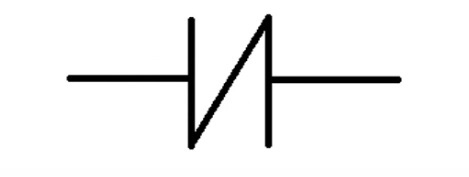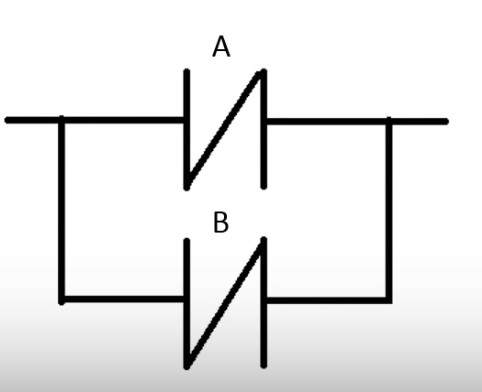# What is Normally Open and Normally Close switch ? How to implement logic gates with NO and NC switch?

Boolean logic is represented by two states.

True = 1 False = 0

This is also known as a digital state.

Normally Open Switch

State 0

When the switch has no information or a reading of ‘false’ or ‘0’ state,then no information can be passed on to logic beyond it.You can see the gap keeps data from passing beyond.When this switch has a state of ‘true’ or ‘1’ then it close and looks like this.

State 1Looking at this switch we can see that data is now “connected” across the center an logic beyond it can be computed.

Normally Closed Switch

This is the opposite of the normally open switch. When this switch has no information or a reading of ‘false’ or ‘0’ state,then the information can be passed on to logic beyond it. when this switch has a state of true or ‘1’ then it opens looks like this.

AND Gate

If A and B are ‘true’ or ‘1’ then output is ‘true’ or ‘1’.

If either A or B is false then output will effectively be stopped and be ‘false’ or ‘0’.

Two or more normally open switches in series equal to an “AND” gate.

OR Gate

if A or B are “true” or “1” then the output is true or ‘1’.

If A and B is false then output will effectively be stopped and be ‘false’ or ‘0’.

Two or more normally open switch in parallel equal to “OR” gate.

NOR Gate

If A and B is ‘false’ or ‘0’ then output is ‘true’ or ‘1’.

If either A OR B is true then the output will effectively be stopped and be ‘false’ or ‘0’.

Two or more normally closed switch in series is equal to NOR gate.

NAND Gate

If either A or B is ‘false’ or ‘0’ then the output is equal to ‘true’ or ‘1’.

If A and B is true then the output will effectively be stopped and be ‘false’ or ‘0’.

Two or more normally close switches in parallel equal to NAND gate.XOR Gate

When A and B are present we want an output to be ‘1’ or ‘true’ when only one of the input is true. If A and B are true then the output is blocked and is in state “0” or ‘false’.

If A is true then B must be false. If B is true then A must be false to have output state = ‘1’.

A normally open gate in series with a normally closed gate while in parallel with the same input with opposite disposition.